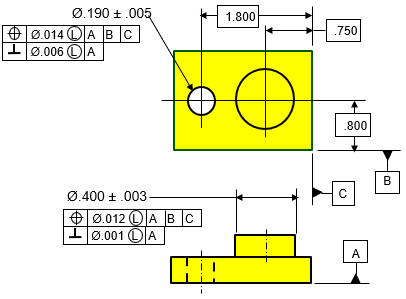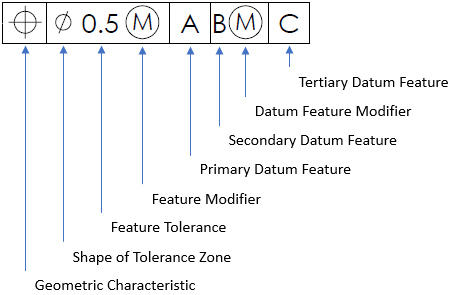## A Hole’s Virtual Size

Answer the following questions based on the figure shown.
1. What is the hole’s LMC size?
2. What is the hole’s Virtual Size relative to Datums ABC?
3. What is the hole’s Virtual Size relative to Datum A?Hint
Breaking down the parts of a feature control frame:Hint 2
The virtual condition is used to analyze the clearance distance between mating parts. It is a constant boundary generated by a combination of a feature of size’s specified MMC or LMC and the geometric tolerance for that material condition.
Breaking down the parts of a feature control frame:The problem statement specifies a Least Material Condition (LMC) feature modifier. A LMC modifier describes a size condition in which the least amount of material exists within its dimensional tolerance. For a hole, LMC is the dimension that produces the largest cutout. Thus,
$$LMC_{hole}=.190+.005=.195$$$The virtual condition is used to analyze the clearance distance between mating parts. It is a constant boundary generated by a combination of a feature of size’s specified MMC or LMC and the geometric tolerance for that material condition. In summary, For an Internal Feature (Hole) with Geo Tol at LMC: • Outer Boundary (OB) = LMC (think largest hole) + geo tol = Virtual Size/Condition For an External Feature (Pin) with Geo Tol at LMC: • Inner Boundary (IB) = LMC (think smallest pin) - geo tol = Virtual Size/Condition where $$geo\:tol$$ is the stated value in the feature control frame. In the feature control frame, the positional tolerance is the one associated with Datums ABC. Thus, the hole’s virtual size is: $$.195+.014=.209$$$
In the feature control frame, the perpendicularity tolerance is the one associated with Datum A only. Thus, the hole’s virtual size is:
$$.195+.006=.201$$\$
1. .195
2. .209
3. .201